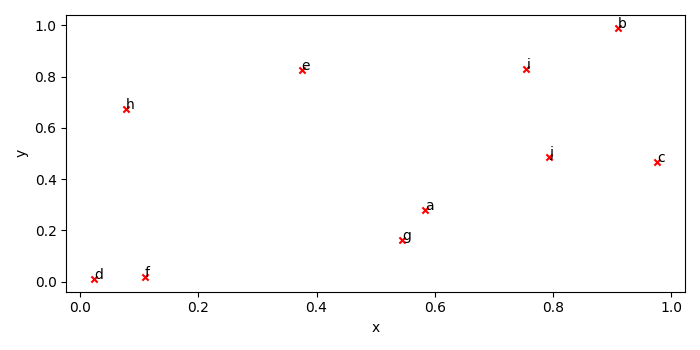# Annotate data points while plotting from Pandas DataFrame

To annotate data points while plotting from pandas data frame, we can take the following steps −

• Create df using DataFrame with x, y and index keys.

• Create a figure and a set of subplots using subplots() method.

• Plot a series of data frame using plot() method, kind='scatter', ax=ax, c='red' and marker='x'.

• To annotate the scatter point with the index value, iterate the data frame.

• To display the figure, use show() method.

## Example

import numpy as np
import pandas as pd
from matplotlib import pyplot as plt
import string
plt.rcParams["figure.figsize"] = [7.00, 3.50]
plt.rcParams["figure.autolayout"] = True
df = pd.DataFrame({'x': np.random.rand(10), 'y': np.random.rand(10)}, index=list(string.ascii_lowercase[:10]))
fig, ax = plt.subplots()
df.plot('x', 'y', kind='scatter', ax=ax, c='red', marker='x')
for k, v in df.iterrows():
ax.annotate(k, v)
plt.show()

## Output